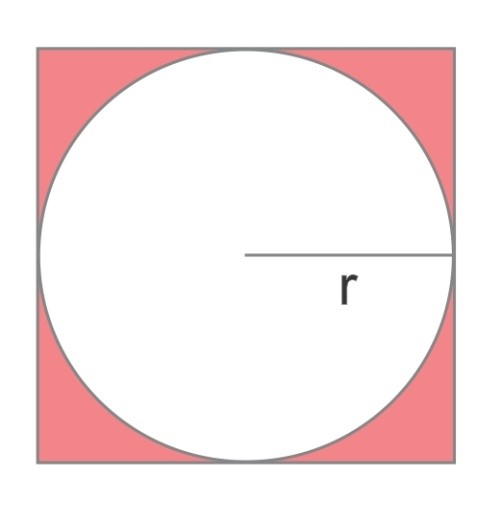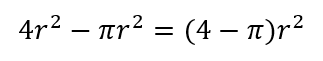# TEAS Mathematics Quiz Answer Explanations

1. Write the expression for three times the sum of twice a number and one minus 6.

Explanation: The expression is three times the sum of twice a number and 1, which is 3(2x+1). Then, 6 is subtracted from this expression.

2. Which of the following inequalities is equivalent to 3 – (1/2)x ≥ 2?

Explanation: To simplify this inequality, subtract 3 from both sides to get: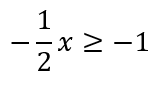Then, multiply both sides by -2 (remembering this flips the direction of the inequality) to get x ≤ 2.

3. 6 is 30% of what number?

Explanation: 30% is 3/10. The number itself must be 10/3 of 6, or (10/3) × 6 = 20.

4. Solve the following equation: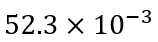Explanation: Multiplying by 10^(-3) means moving the decimal point three places to the left, putting in zeroes as necessary.

5. A closet is filled with red, blue, and green shirts. If 1/3 of the shirts are green and 2/5 are red, what fraction of the shirts are blue?

Explanation: The total fraction taken up by green and red shirts will be: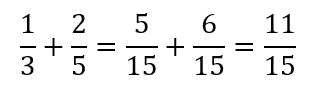The remaining fraction is: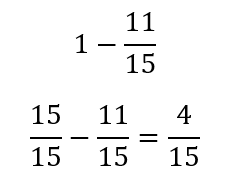6. On Monday, Robert mopped the floor in 4 hours. On Tuesday, he did it in 3 hours. If on Monday, his average rate of mopping was p sq. ft. per hour, what was his average rate on Tuesday?

Answer: (4/3)p sq. ft. per hour
Explanation: Robert accomplished his task on Tuesday in 3/4 the time compared to Monday. He must have worked 4/3 as fast.

7. A rectangle has a length that is 5 feet longer than three times its width. If the perimeter is 90 feet, what is the length in feet?

Explanation: Denote the width as w and the length as l. Then, l=3w+5. The perimeter is 2w+2l = 90. Substituting the first expression for l into the second equation yields 2(3w+5)+2w = 90, or 8w = 80, so w = 10. Putting this into the first equation, it yields: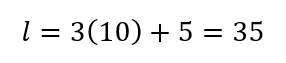8. A company invests \$50,000 in a building where they can produce saws. If the cost of producing one saw is \$40, then which function expresses the amount of money the company pays? The variable y is the money paid and x is the number of saws produced.

Explanation: For manufacturing costs, there is a linear relationship between the cost to the company and the number produced, with a y-intercept given by the base cost of acquiring the means of production, and a slope given by the cost to produce one unit. In this case, that base cost is \$50,000, while the cost per unit is \$40. So, y = 40x+50,000.

9. How do you solve V = lwh for h?